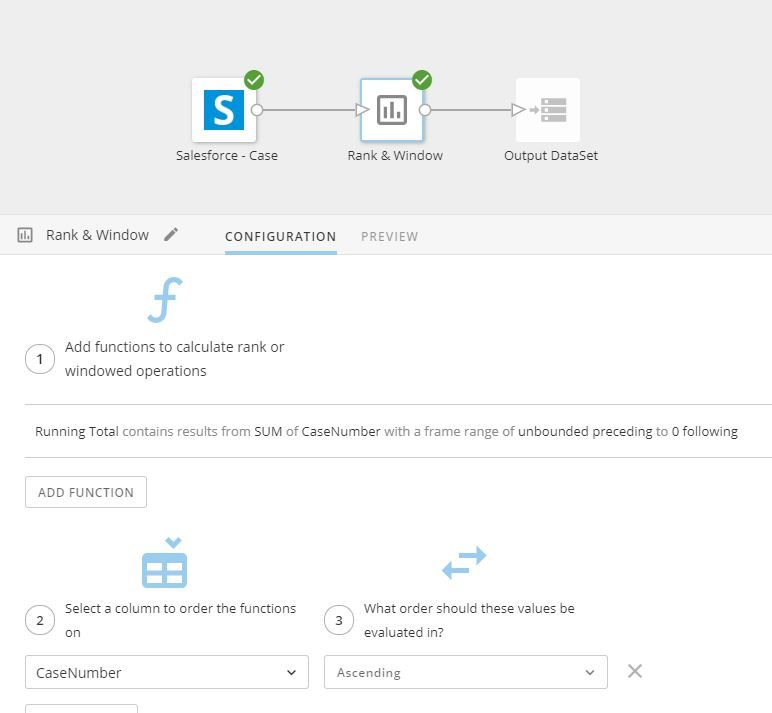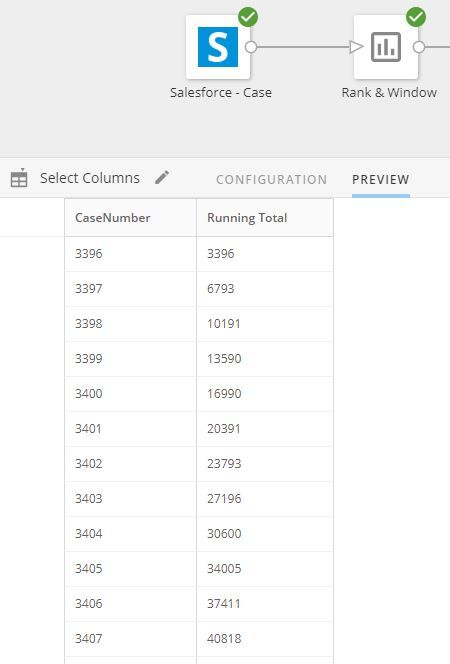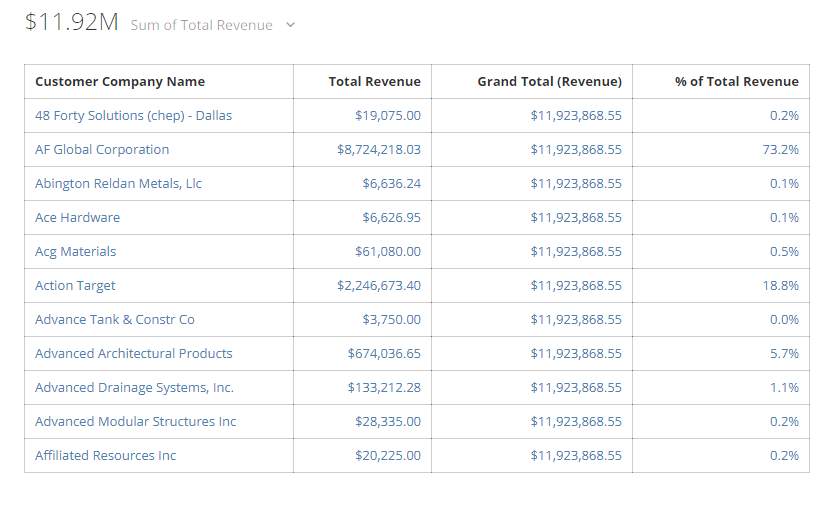# cumulative sum

Is it possible to have a cumulative sum for values in one column to show in another so that the final today would be in the last row? ex. in the attached screenshot If I wanted to have Total Revenue cumulatively sum and show each new value in a column next to it so the in the first row of 'Cumulative Sum' would be 19075 and the second row would be 19075+8724218.03, in the third would be 19075+8724218.03+66363.24, etc

My hope is then if I can generate this then create another beastmode to have the max of the column cumulative sum so that I would just have the grand total

thanks

•ContributorI'm not sure if you can do it in a beastmode or not but you can achieve something like that you're looking for in an ETL.

Use the Rank & Window block with a function of SUM based on your Total Revenue field and make it unbounded with a preceeding of 0.When I look just at those columns you can see it's a running total.**Make sure to like any users posts that helped you and accept the ones who solved your issue.**
•Coach@meadow_ryan - I'm trying to understand your use case.  You want to calculate a running total so that you can take the max() of that field to get a grand total?

Unless there is more to this, why not just sum the column?

If the intention is to understand what percentage of total revenue is coming from each company, you can to this using beastmodes.

For `Grand Total (Revenue)`

`(sum(`Total Revenue`) over ())`

for % of revenue

``Total Revenue` / (sum(`Total Revenue`) over ())`

This will give you something like this:The advantage to doing this in the beastmode is that the grand total and the percentage will recalculate as you apply any filters to the card

“There is a superhero in all of us, we just need the courage to put on the cape.” -Superman
• I added the following to my card and the card doesn't display any data now

`Total Revenue`/(sum(`Total Revenue`) over ())

• entering as it looks in the attached screenshot and its not taking it?

•Coachis `Total Revenue` ever null?

try

`IFNULL(`Total Revenue`,0) / (SUM(IFNULL(`Total Revenue`,0)) OVER())`

“There is a superhero in all of us, we just need the courage to put on the cape.” -Superman
• don't believe it ever will be, but when I add the beast mode function receive the following in the screenshot

•CoachYou need to make sure that none of the other fields are aggregate functions as well.

I'm guessing that =Profit is an aggregate field.  Try taking it off or removing the aggregate from the beastmode formula

“There is a superhero in all of us, we just need the courage to put on the cape.” -Superman
• =Profit is

SUM(`Total Revenue` - ABS(`Total Cost`))

•Coachchange it to:

``Total Revenue` - ABS(`Total Cost`)`

“There is a superhero in all of us, we just need the courage to put on the cape.” -Superman
• getting the following now

•Coachchange the field to display as a percent with 2 decimals

“There is a superhero in all of us, we just need the courage to put on the cape.” -Superman
This discussion has been closed.